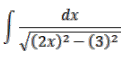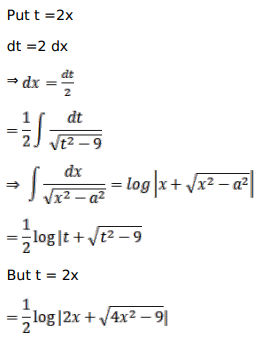# Mark against the correct answer in each of the following:Question:

Mark $(\sqrt{)}$ against the correct answer in each of the following:

$\int \frac{d x}{\sqrt{4 x^{2}-9}}=?$

A. $\frac{1}{2} \log \left|2 x+\sqrt{4 x^{2}-9}\right|+C$

B. $\frac{1}{4} \log \left|x+\sqrt{4 x^{2}-9}\right|+C$

C. $\log \left|2 x+\sqrt{4 x^{2}-9}\right|+C$

D. none of these

Solution: